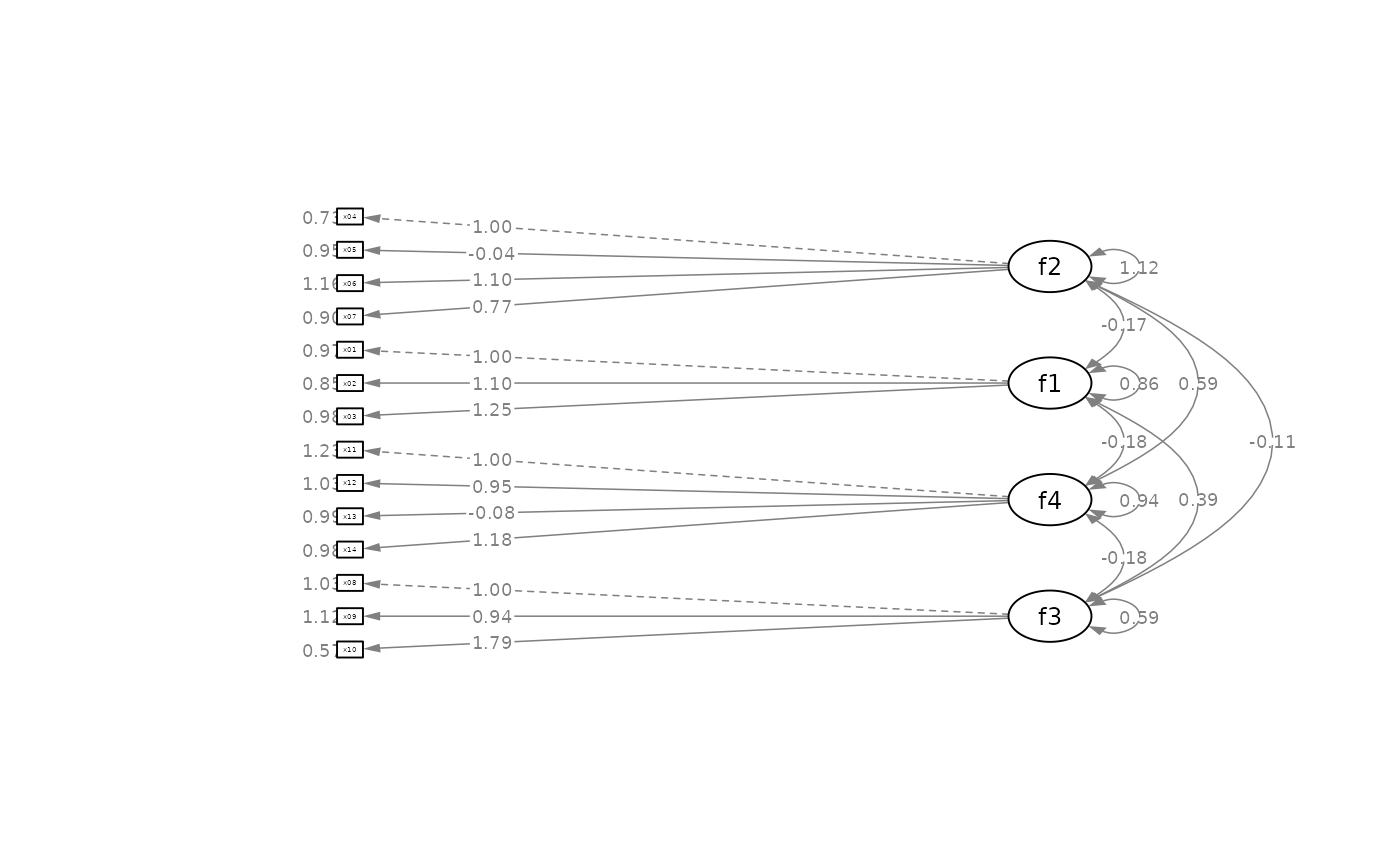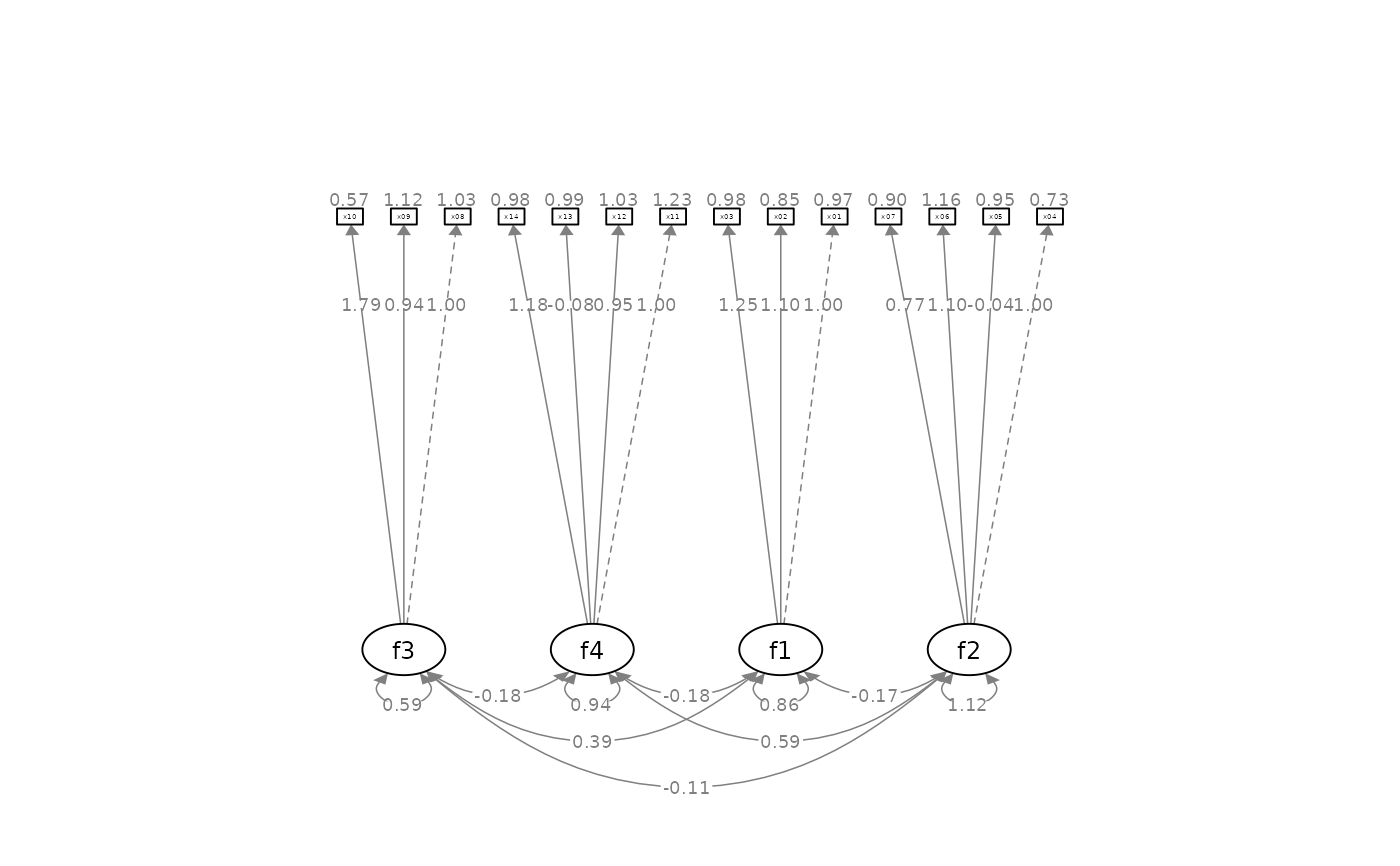Configure the layout of factors and adjust other aspects of a CFA graph by semPaths.

set_cfa_layout(
semPaths_plot,
indicator_order = NULL,
indicator_factor = NULL,
fcov_curve = 0.4,
point_to = "down"
)

## Arguments

semPaths_plot

A qgraph::qgraph object generated by semPaths, or a similar qgraph object modified by other semptools functions.

indicator_order

A string vector of the indicators. The order of the names is the order of the indicators in the graph, when they are drawn on the bottom of the graph. The indicators should be grouped by the factors on which they load on. For example, if x1, x2, x4 load on f2, and x3, x5, x6 load on f1, then vector should be either c("x1", "x2", "x4", "x3", "x5", "x6") or c("x3", "x5", "x6", "x1", "x2", "x4"). Indicators within a group can be ordered in any way.

indicator_factor

A string vector of the same length of the indicator order, storing the name of the factor for which each of the indicator in indicator_factor loads on. For example, if x1, x2, x4 load on f2, and x3, x5, x6 load on f1, and indicator_order is c("x3", "x5", "x6", "x1", "x2", "x4"), then indicator_factor should be c("f2", "f2", "f2", "f1", "f1", "f1").

fcov_curve

A number used to set the curvature of the inter-factor covariances. Default is .4.

point_to

Can be "down", "left", "up", or "right". Specify the direction that the factors "point" to the indicators. Default is "down".

## Value

A qgraph::qgraph based on the original one, with various aspects of the model modified.

## Details

Modify a qgraph::qgraph object generated by semPaths based on a confirmatory factor analysis model.

## Examples

library(lavaan)
library(semPlot)
mod <-
'f1 =~ x01 + x02 + x03
f2 =~ x04 + x05 + x06 + x07
f3 =~ x08 + x09 + x10
f4 =~ x11 + x12 + x13 + x14
'
fit_cfa <- lavaan::sem(mod, cfa_example)
lavaan::parameterEstimates(fit_cfa)[, c("lhs", "op", "rhs", "est", "pvalue")]
#>    lhs op rhs    est pvalue
#> 1   f1 =~ x01  1.000     NA
#> 2   f1 =~ x02  1.097  0.000
#> 3   f1 =~ x03  1.247  0.000
#> 4   f2 =~ x04  1.000     NA
#> 5   f2 =~ x05 -0.040  0.587
#> 6   f2 =~ x06  1.098  0.000
#> 7   f2 =~ x07  0.771  0.000
#> 8   f3 =~ x08  1.000     NA
#> 9   f3 =~ x09  0.937  0.000
#> 10  f3 =~ x10  1.785  0.000
#> 11  f4 =~ x11  1.000     NA
#> 12  f4 =~ x12  0.949  0.000
#> 13  f4 =~ x13 -0.077  0.356
#> 14  f4 =~ x14  1.184  0.000
#> 15 x01 ~~ x01  0.969  0.000
#> 16 x02 ~~ x02  0.853  0.000
#> 17 x03 ~~ x03  0.976  0.000
#> 18 x04 ~~ x04  0.725  0.000
#> 19 x05 ~~ x05  0.954  0.000
#> 20 x06 ~~ x06  1.161  0.000
#> 21 x07 ~~ x07  0.903  0.000
#> 22 x08 ~~ x08  1.026  0.000
#> 23 x09 ~~ x09  1.119  0.000
#> 24 x10 ~~ x10  0.566  0.009
#> 25 x11 ~~ x11  1.231  0.000
#> 26 x12 ~~ x12  1.032  0.000
#> 27 x13 ~~ x13  0.990  0.000
#> 28 x14 ~~ x14  0.985  0.000
#> 29  f1 ~~  f1  0.855  0.000
#> 30  f2 ~~  f2  1.119  0.000
#> 31  f3 ~~  f3  0.585  0.000
#> 32  f4 ~~  f4  0.943  0.000
#> 33  f1 ~~  f2 -0.173  0.059
#> 34  f1 ~~  f3  0.387  0.000
#> 35  f1 ~~  f4 -0.178  0.041
#> 36  f2 ~~  f3 -0.112  0.132
#> 37  f2 ~~  f4  0.593  0.000
#> 38  f3 ~~  f4 -0.181  0.014
p <- semPaths(fit_cfa, whatLabels="est",
sizeMan = 2.5,
nCharNodes = 0, nCharEdges = 0,
edge.width = 0.8, node.width = 0.7,
edge.label.cex = 0.6,
style = "ram",
mar = c(10,10,10,10))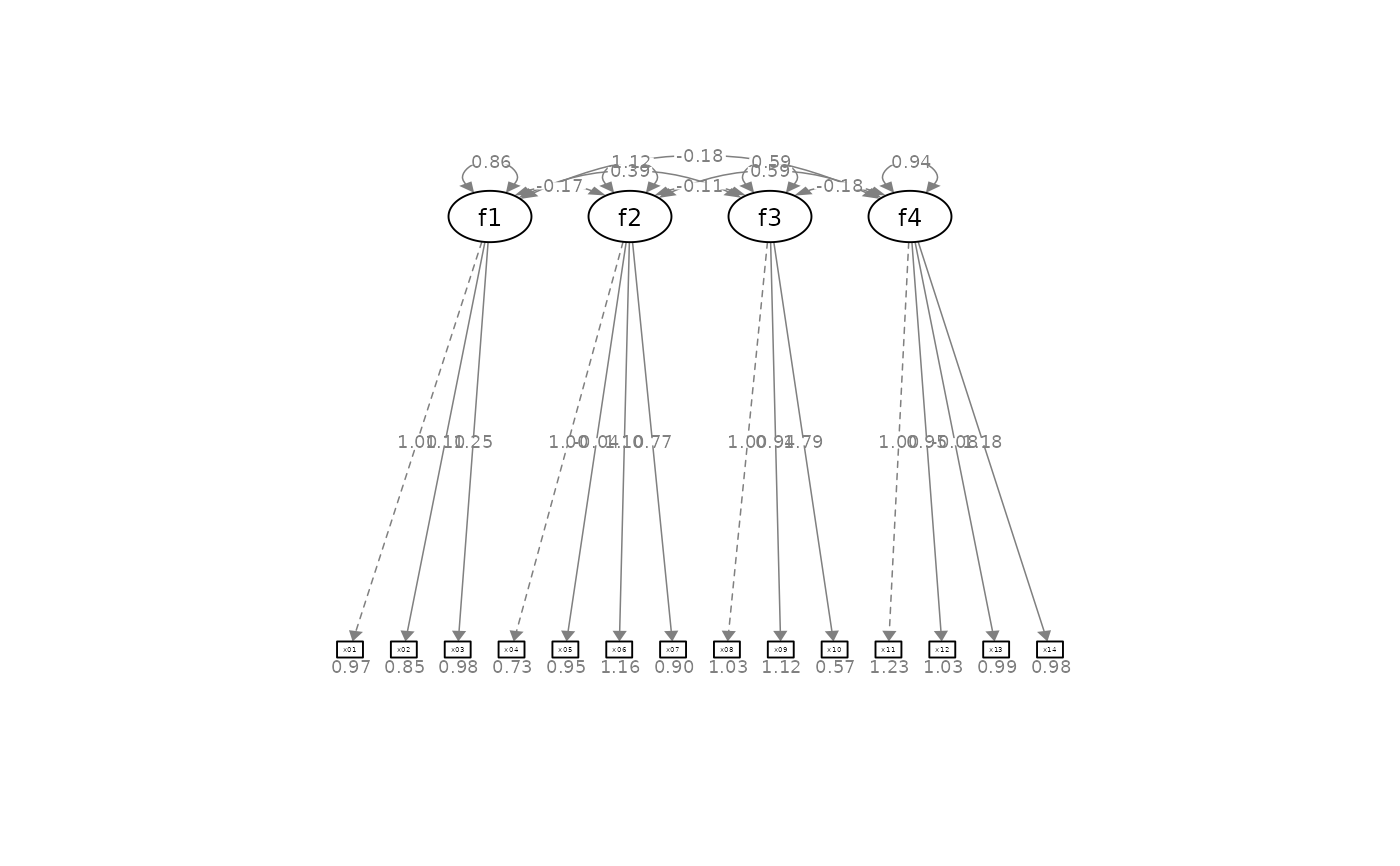indicator_order  <- c("x04", "x05", "x06", "x07", "x01", "x02", "x03", "x11",
"x12", "x13", "x14", "x08", "x09", "x10")
indicator_factor <- c( "f2",  "f2",  "f2",  "f2",  "f1",  "f1",  "f1",  "f4",
"f4",  "f4",  "f4",  "f3",  "f3",  "f3")
p2 <- set_cfa_layout(p, indicator_order,
indicator_factor,
fcov_curve = 1.5,
plot(p2)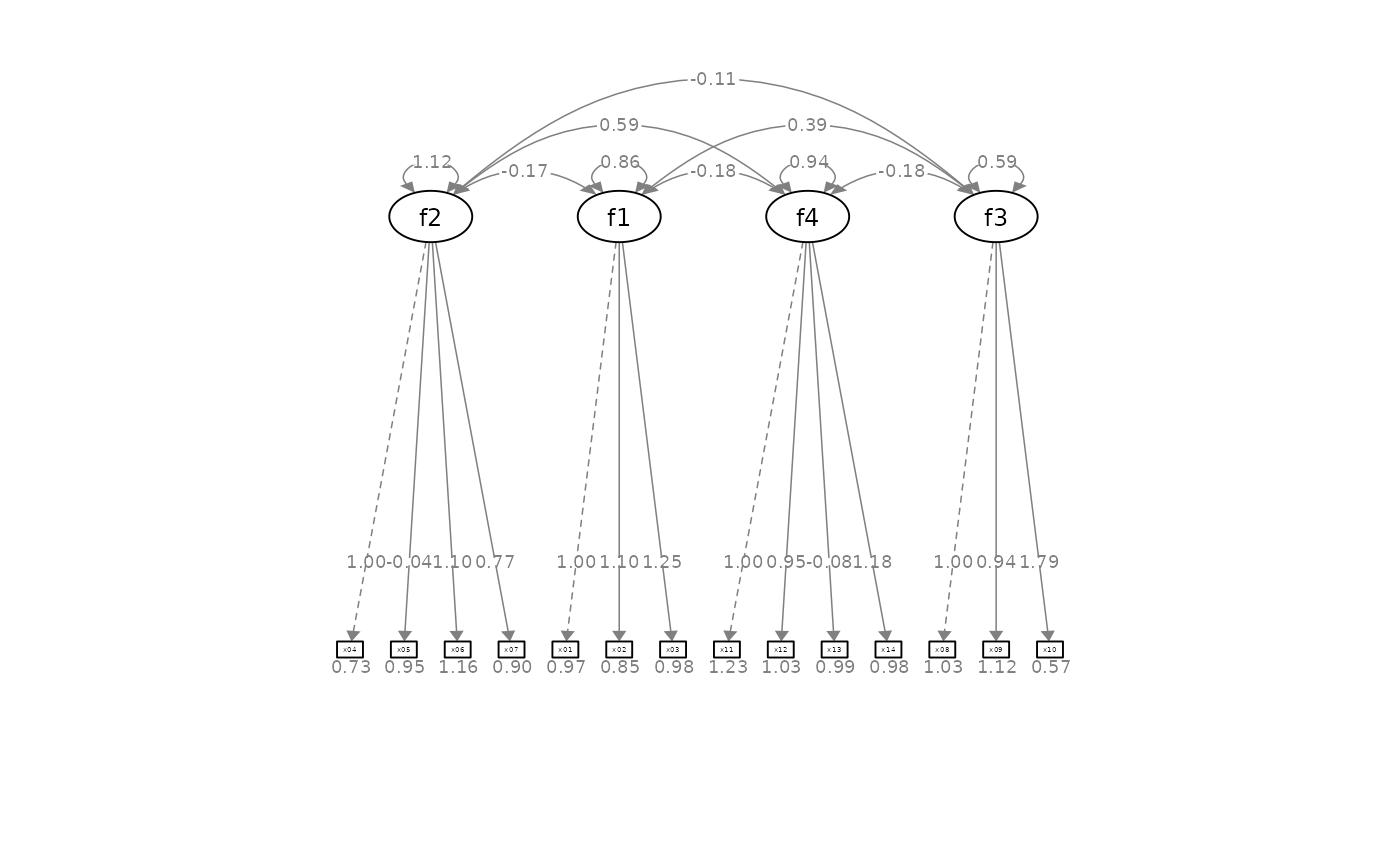p2 <- set_cfa_layout(p, indicator_order,
indicator_factor,
fcov_curve = 1.5,
point_to = "left")
plot(p2)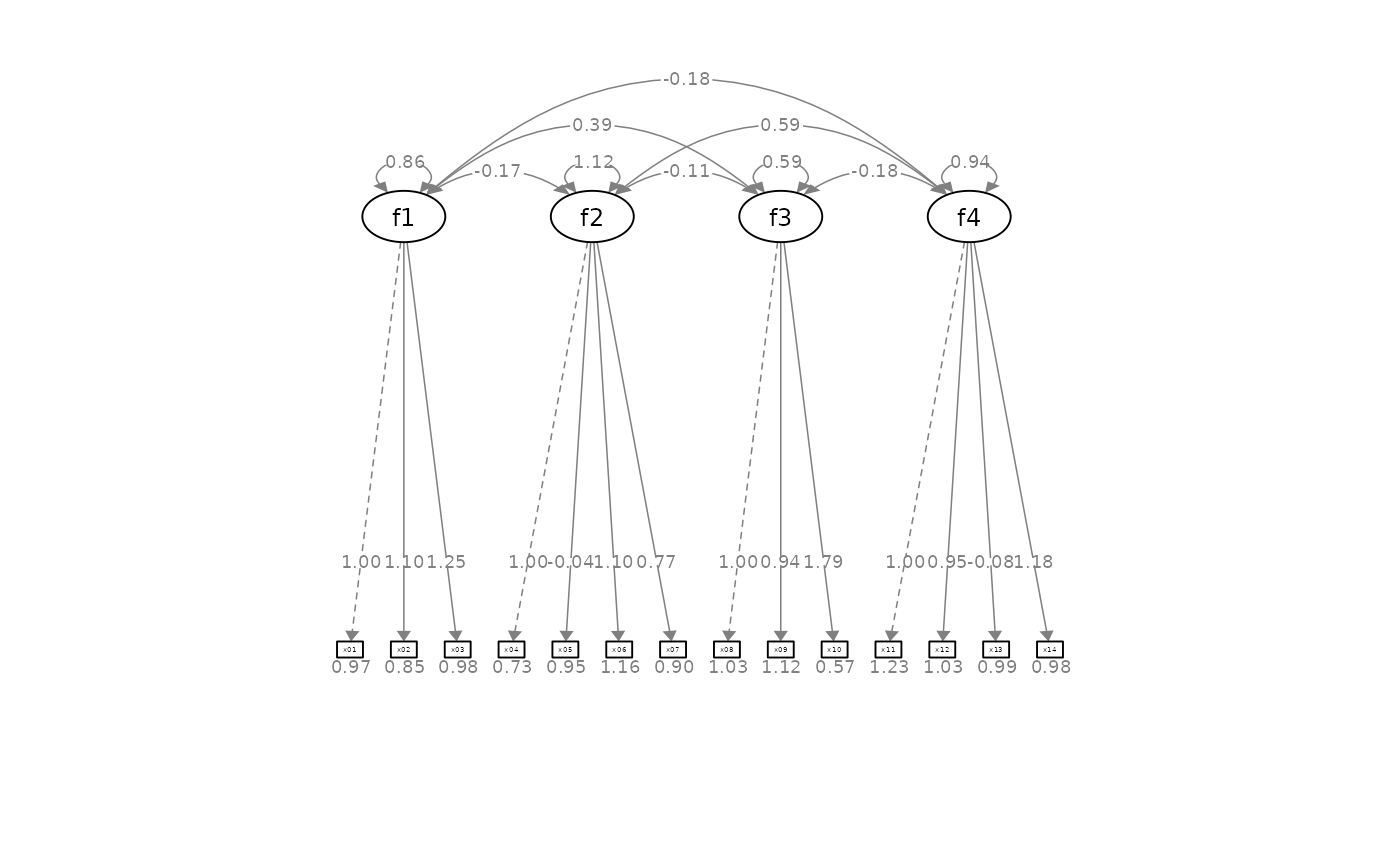p2 <- set_cfa_layout(p, indicator_order,
indicator_factor,
fcov_curve = 1.5,